# Small magnetic loop antenna

The magnetic loop antenna consists of two circles: one large one and a smaller one in the larger one. The circumference should be between 0.1 and 0.25 wavelength for optimal efficiency.

### Formulas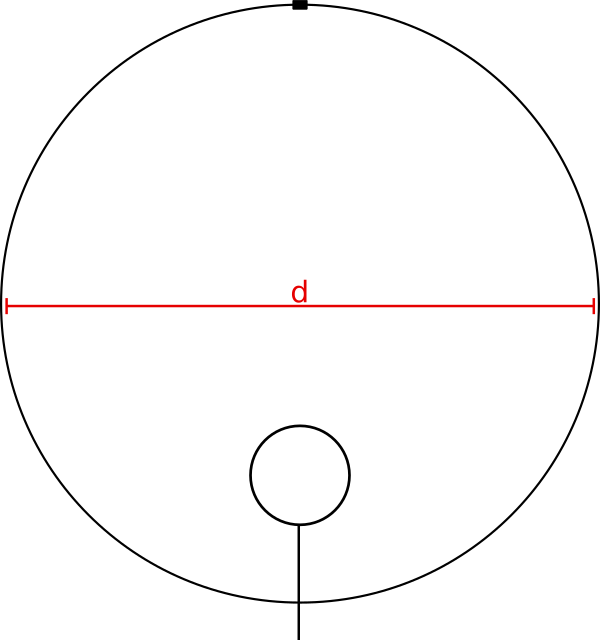Schematic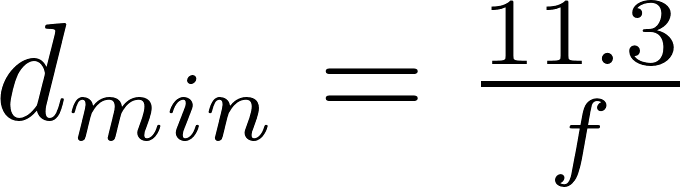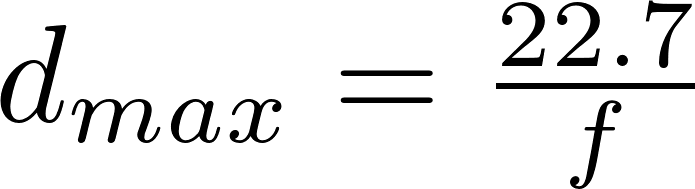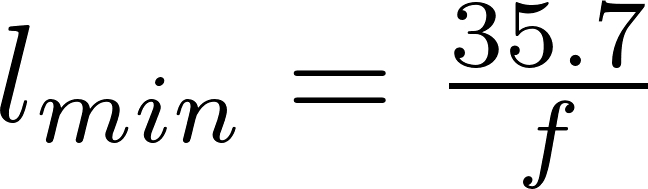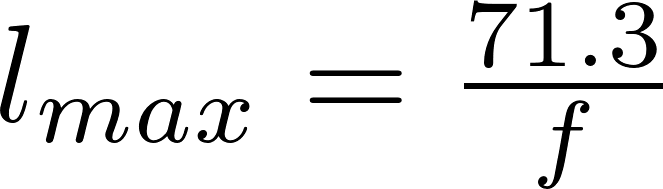l is the symbol for length and is measured in meter (m).
f is the frequency in megahertz (MHz).
d min is the symbol for minimum diameter and is measured in meter (m).
d max is the symbol for maximum diameter and is measured in meter (m).
l min is the symbol for minimum conductor length and is measured in meter (m).
l max is the symbol for maxinum conductor length and is measured in meter (m).